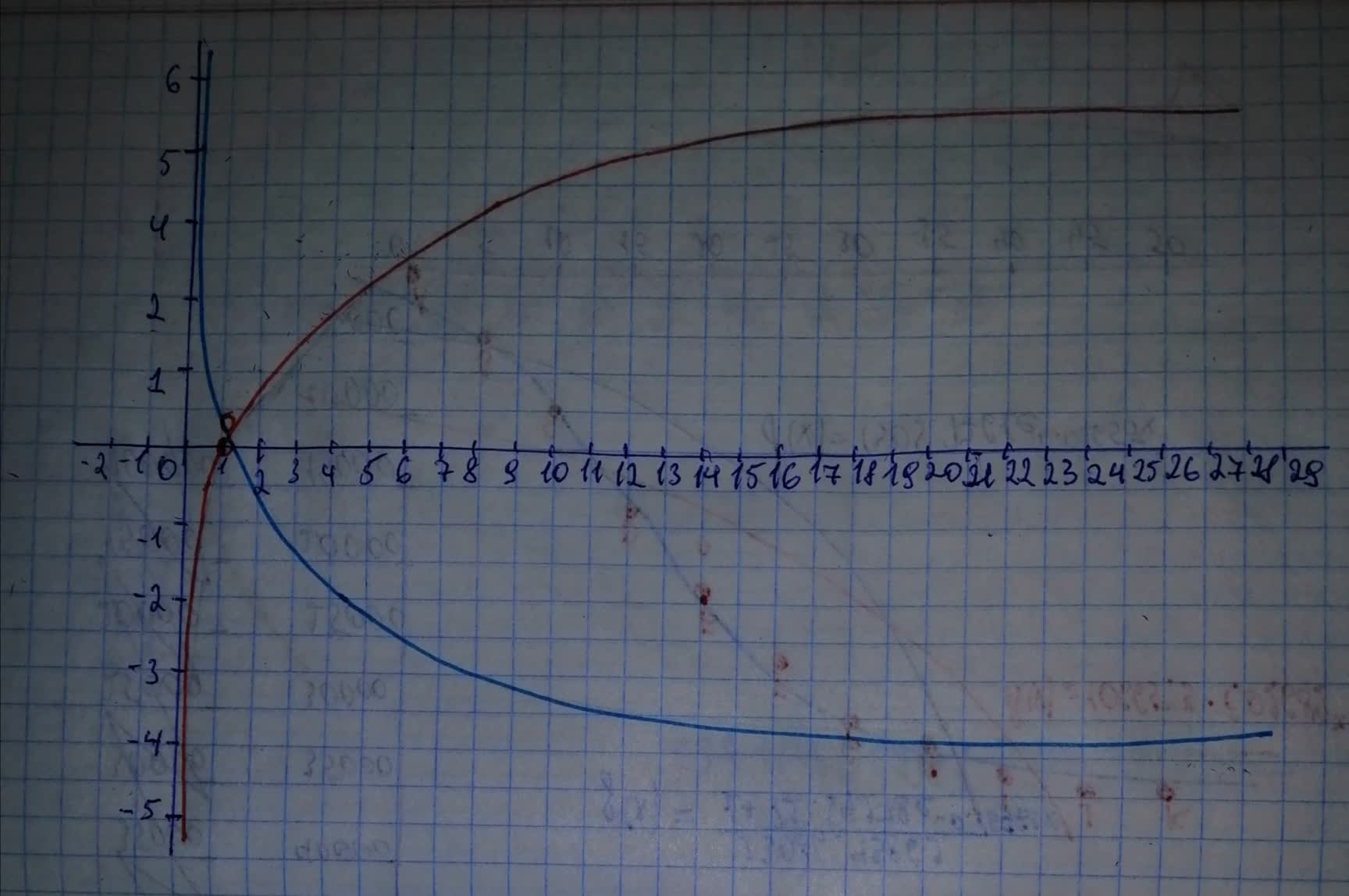ringearV

2021-02-11

For .
a) Use transformations of the graphs of $y={\mathrm{log}}_{2}x$ and $y={\mathrm{log}}_{3}x$ o graph the given functions.
b) Write the domain and range in interval notation.
c) Write an equation of the asymptote.davonliefI

Step 1
a) Start from the graph of the parent function $f\left(x\right)={\mathrm{log}}_{2}x$.
As we can see the given function can be expressed in terms of the parent function f as
This indicates that the graph of the function will be the same as the graph of the parent function $f\left(x\right)={\mathrm{log}}_{2}x$ reflected through the x-axis.
See the graphs in the picture below:Step 2
b) The domain of the function is the interval:
The range of the function is the interval
c) The vertical asymptote of the graph of this function is the line $x=0$

Do you have a similar question?